# Graphs: Finding shortest paths

28 May 2013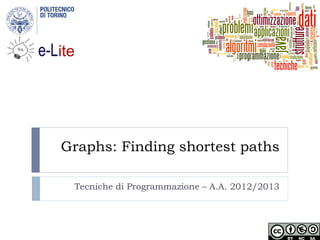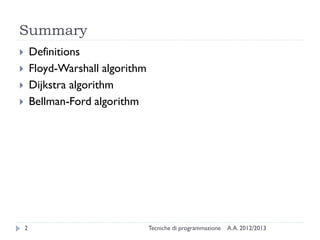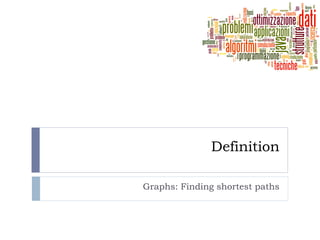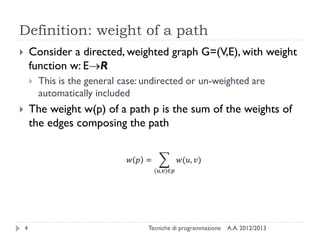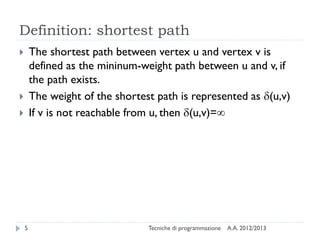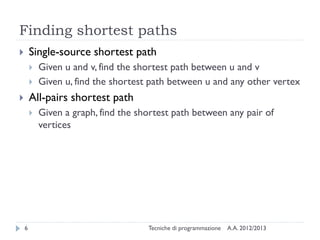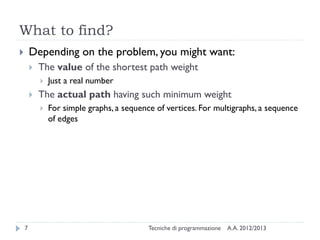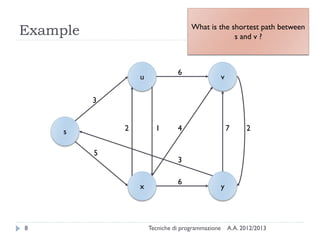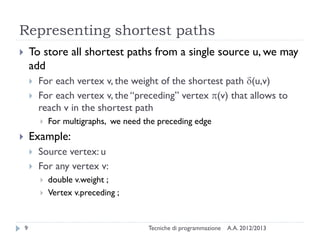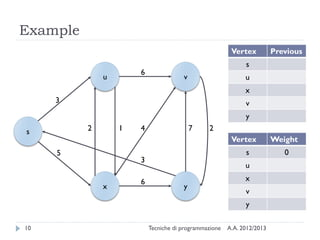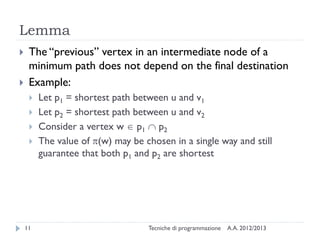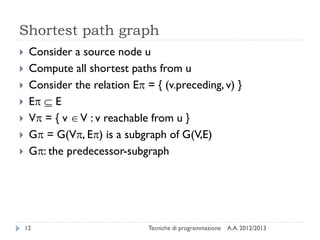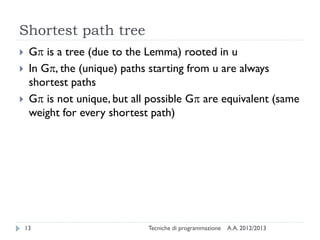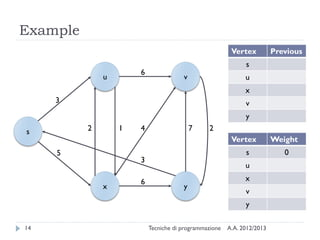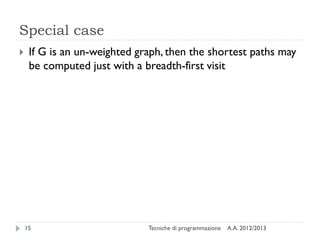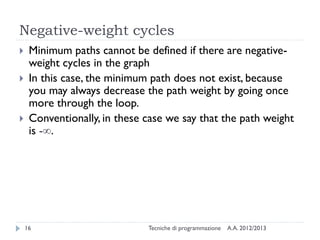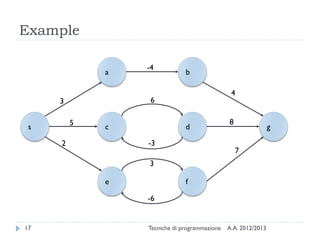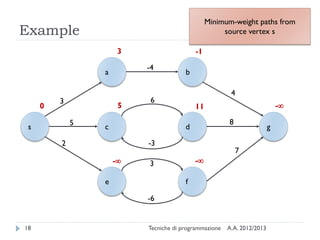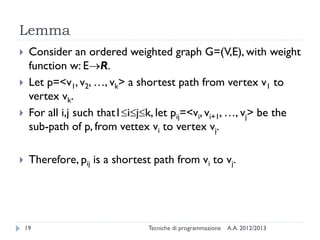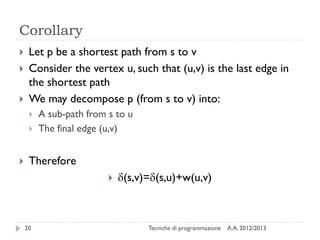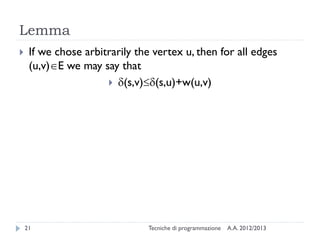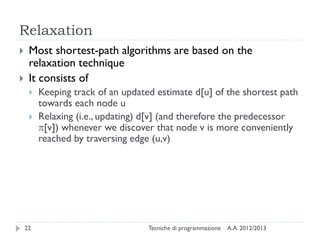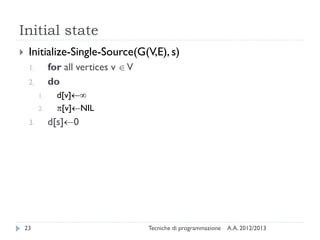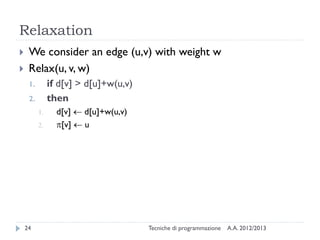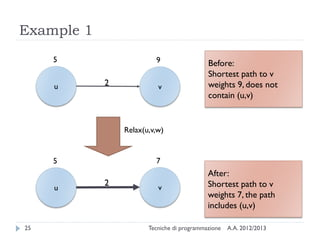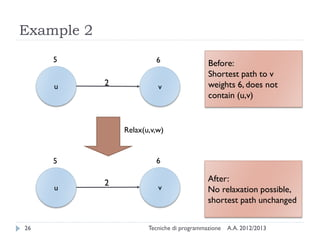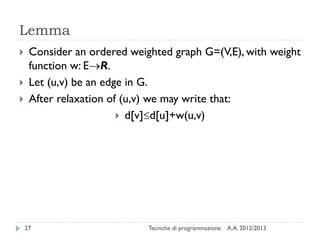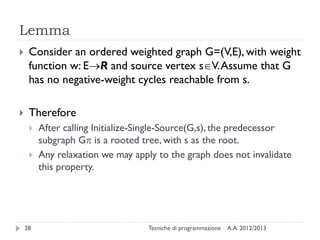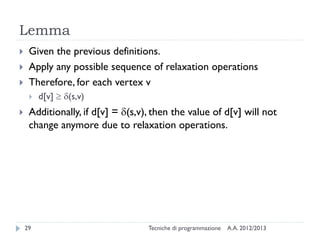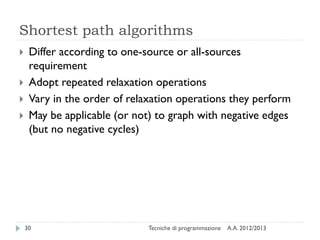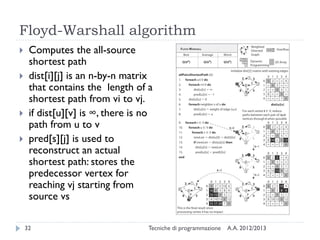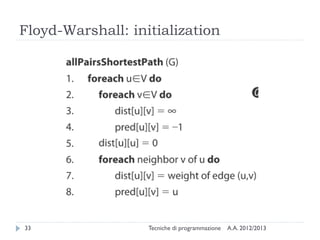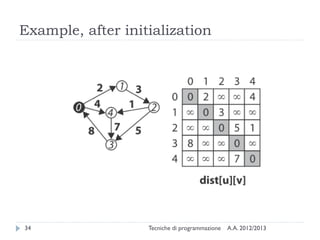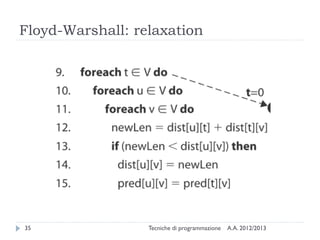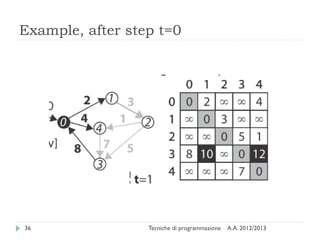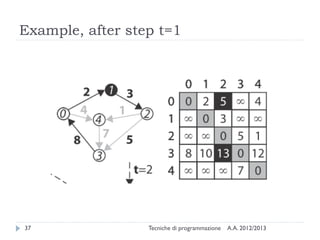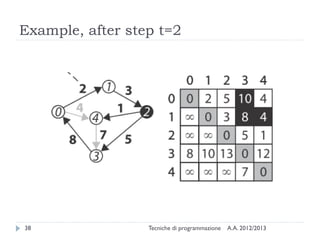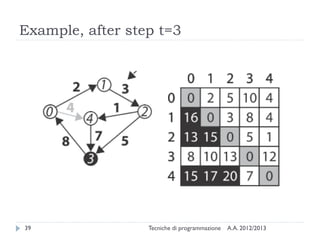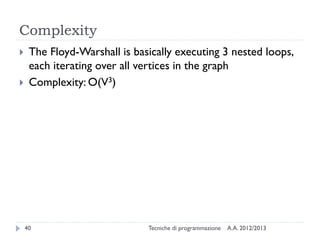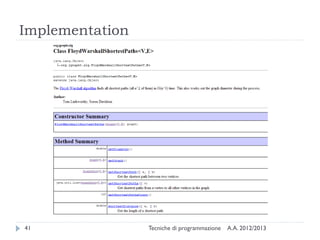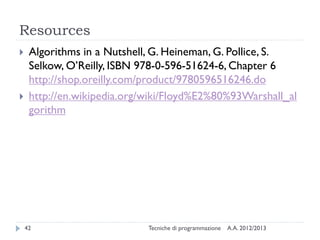1 sur 43

### Graphs: Finding shortest paths

• 1. Graphs: Finding shortest paths Tecniche di Programmazione – A.A. 2012/2013
• 2. Summary A.A. 2012/2013Tecniche di programmazione2  Definitions  Floyd-Warshall algorithm  Dijkstra algorithm  Bellman-Ford algorithm
• 3. Definition Graphs: Finding shortest paths
• 4. Definition: weight of a path A.A. 2012/2013Tecniche di programmazione4  Consider a directed, weighted graph G=(V,E), with weight function w: ER  This is the general case: undirected or un-weighted are automatically included  The weight w(p) of a path p is the sum of the weights of the edges composing the path 𝑤 𝑝 = 𝑤(𝑢, 𝑣) (𝑢,𝑣)∈𝑝
• 5. Definition: shortest path A.A. 2012/2013Tecniche di programmazione5  The shortest path between vertex u and vertex v is defined as the mininum-weight path between u and v, if the path exists.  The weight of the shortest path is represented as d(u,v)  If v is not reachable from u, then d(u,v)=
• 6. Finding shortest paths A.A. 2012/2013Tecniche di programmazione6  Single-source shortest path  Given u and v, find the shortest path between u and v  Given u, find the shortest path between u and any other vertex  All-pairs shortest path  Given a graph, find the shortest path between any pair of vertices
• 7. What to find? A.A. 2012/2013Tecniche di programmazione7  Depending on the problem, you might want:  The value of the shortest path weight  Just a real number  The actual path having such minimum weight  For simple graphs, a sequence of vertices. For multigraphs, a sequence of edges
• 8. Example A.A. 2012/2013Tecniche di programmazione8 u v s x y 3 5 6 4 3 12 7 2 6 What is the shortest path between s and v ?
• 9. Representing shortest paths A.A. 2012/2013Tecniche di programmazione9  To store all shortest paths from a single source u, we may add  For each vertex v, the weight of the shortest path d(u,v)  For each vertex v, the “preceding” vertex p(v) that allows to reach v in the shortest path  For multigraphs, we need the preceding edge  Example:  Source vertex: u  For any vertex v:  double v.weight ;  Vertex v.preceding ;
• 10. Example A.A. 2012/2013Tecniche di programmazione10 u v s x y 3 5 6 4 3 12 7 2 6 Vertex Previous s u x v y Vertex Weight s 0 u x v y
• 11. Lemma A.A. 2012/2013Tecniche di programmazione11  The “previous” vertex in an intermediate node of a minimum path does not depend on the final destination  Example:  Let p1 = shortest path between u and v1  Let p2 = shortest path between u and v2  Consider a vertex w  p1  p2  The value of p(w) may be chosen in a single way and still guarantee that both p1 and p2 are shortest
• 12. Shortest path graph A.A. 2012/2013Tecniche di programmazione12  Consider a source node u  Compute all shortest paths from u  Consider the relation Ep = { (v.preceding, v) }  Ep  E  Vp = { v V : v reachable from u }  Gp = G(Vp, Ep) is a subgraph of G(V,E)  Gp: the predecessor-subgraph
• 13. Shortest path tree A.A. 2012/2013Tecniche di programmazione13  Gp is a tree (due to the Lemma) rooted in u  In Gp, the (unique) paths starting from u are always shortest paths  Gp is not unique, but all possible Gp are equivalent (same weight for every shortest path)
• 14. Example A.A. 2012/2013Tecniche di programmazione14 u v s x y 3 5 6 4 3 12 7 2 6 Vertex Previous s u x v y Vertex Weight s 0 u x v y
• 15. Special case A.A. 2012/2013Tecniche di programmazione15  If G is an un-weighted graph, then the shortest paths may be computed just with a breadth-first visit
• 16. A.A. 2012/2013Tecniche di programmazione16 Negative-weight cycles  Minimum paths cannot be defined if there are negative- weight cycles in the graph  In this case, the minimum path does not exist, because you may always decrease the path weight by going once more through the loop.  Conventionally, in these case we say that the path weight is -.
• 17. A.A. 2012/2013Tecniche di programmazione17 Example a b s c d g e f 3 5 2 -4 4 8 7 6 -3 3 -6
• 18. A.A. 2012/2013Tecniche di programmazione18 Example a b s c d g e f 3 5 2 -4 4 8 7 6 -3 3 -6 0 3 -1 - -- 5 11 Minimum-weight paths from source vertex s
• 19. A.A. 2012/2013Tecniche di programmazione19 Lemma  Consider an ordered weighted graph G=(V,E), with weight function w: ER.  Let p=<v1, v2, …, vk> a shortest path from vertex v1 to vertex vk.  For all i,j such that1ijk, let pij=<vi, vi+1, …, vj> be the sub-path of p, from vettex vi to vertex vj.  Therefore, pij is a shortest path from vi to vj.
• 20. A.A. 2012/2013Tecniche di programmazione20 Corollary  Let p be a shortest path from s to v  Consider the vertex u, such that (u,v) is the last edge in the shortest path  We may decompose p (from s to v) into:  A sub-path from s to u  The final edge (u,v)  Therefore  d(s,v)=d(s,u)+w(u,v)
• 21. A.A. 2012/2013Tecniche di programmazione21 Lemma  If we chose arbitrarily the vertex u, then for all edges (u,v)E we may say that  d(s,v)d(s,u)+w(u,v)
• 22. A.A. 2012/2013Tecniche di programmazione22 Relaxation  Most shortest-path algorithms are based on the relaxation technique  It consists of  Keeping track of an updated estimate d[u] of the shortest path towards each node u  Relaxing (i.e., updating) d[v] (and therefore the predecessor p[v]) whenever we discover that node v is more conveniently reached by traversing edge (u,v)
• 23. A.A. 2012/2013Tecniche di programmazione23 Initial state  Initialize-Single-Source(G(V,E), s) 1. for all vertices v V 2. do 1. d[v] 2. p[v]NIL 3. d[s]0
• 24. A.A. 2012/2013Tecniche di programmazione24 Relaxation  We consider an edge (u,v) with weight w  Relax(u, v, w) 1. if d[v] > d[u]+w(u,v) 2. then 1. d[v]  d[u]+w(u,v) 2. p[v]  u
• 25. A.A. 2012/2013Tecniche di programmazione25 Example 1 u v 5 9 2 u v 5 7 2 Relax(u,v,w) Before: Shortest path to v weights 9, does not contain (u,v) After: Shortest path to v weights 7, the path includes (u,v)
• 26. A.A. 2012/2013Tecniche di programmazione26 Example 2 u v 5 6 2 u v 5 6 2 Relax(u,v,w) Before: Shortest path to v weights 6, does not contain (u,v) After: No relaxation possible, shortest path unchanged
• 27. A.A. 2012/2013Tecniche di programmazione27 Lemma  Consider an ordered weighted graph G=(V,E), with weight function w: ER.  Let (u,v) be an edge in G.  After relaxation of (u,v) we may write that:  d[v]d[u]+w(u,v)
• 28. Lemma A.A. 2012/2013Tecniche di programmazione28  Consider an ordered weighted graph G=(V,E), with weight function w: ER and source vertex sV.Assume that G has no negative-weight cycles reachable from s.  Therefore  After calling Initialize-Single-Source(G,s), the predecessor subgraph Gp is a rooted tree, with s as the root.  Any relaxation we may apply to the graph does not invalidate this property.
• 29. A.A. 2012/2013Tecniche di programmazione29 Lemma  Given the previous definitions.  Apply any possible sequence of relaxation operations  Therefore, for each vertex v  d[v]  d(s,v)  Additionally, if d[v] = d(s,v), then the value of d[v] will not change anymore due to relaxation operations.
• 30. Shortest path algorithms A.A. 2012/2013Tecniche di programmazione30  Differ according to one-source or all-sources requirement  Adopt repeated relaxation operations  Vary in the order of relaxation operations they perform  May be applicable (or not) to graph with negative edges (but no negative cycles)
• 31. Floyd-Warshall algorithm Graphs: Finding shortest paths
• 32. Floyd-Warshall algorithm A.A. 2012/2013Tecniche di programmazione32  Computes the all-source shortest path  dist[i][j] is an n-by-n matrix that contains the length of a shortest path from vi to vj.  if dist[u][v] is ∞, there is no path from u to v  pred[s][j] is used to reconstruct an actual shortest path: stores the predecessor vertex for reaching vj starting from source vs
• 33. Floyd-Warshall: initialization A.A. 2012/2013Tecniche di programmazione33
• 34. Example, after initialization A.A. 2012/2013Tecniche di programmazione34
• 35. Floyd-Warshall: relaxation A.A. 2012/2013Tecniche di programmazione35
• 36. Example, after step t=0 A.A. 2012/2013Tecniche di programmazione36
• 37. Example, after step t=1 A.A. 2012/2013Tecniche di programmazione37
• 38. Example, after step t=2 A.A. 2012/2013Tecniche di programmazione38
• 39. Example, after step t=3 A.A. 2012/2013Tecniche di programmazione39
• 40. Complexity A.A. 2012/2013Tecniche di programmazione40  The Floyd-Warshall is basically executing 3 nested loops, each iterating over all vertices in the graph  Complexity: O(V3)
• 41. Implementation A.A. 2012/2013Tecniche di programmazione41
• 42. Resources A.A. 2012/2013Tecniche di programmazione42  Algorithms in a Nutshell, G. Heineman, G. Pollice, S. Selkow, O’Reilly, ISBN 978-0-596-51624-6, Chapter 6 http://shop.oreilly.com/product/9780596516246.do  http://en.wikipedia.org/wiki/Floyd%E2%80%93Warshall_al gorithm
• 43. Licenza d’uso A.A. 2012/2013Tecniche di programmazione43  Queste diapositive sono distribuite con licenza Creative Commons “Attribuzione - Non commerciale - Condividi allo stesso modo (CC BY-NC-SA)”  Sei libero:  di riprodurre, distribuire, comunicare al pubblico, esporre in pubblico, rappresentare, eseguire e recitare quest'opera  di modificare quest'opera  Alle seguenti condizioni:  Attribuzione — Devi attribuire la paternità dell'opera agli autori originali e in modo tale da non suggerire che essi avallino te o il modo in cui tu usi l'opera.  Non commerciale — Non puoi usare quest'opera per fini commerciali.  Condividi allo stesso modo — Se alteri o trasformi quest'opera, o se la usi per crearne un'altra, puoi distribuire l'opera risultante solo con una licenza identica o equivalente a questa.  http://creativecommons.org/licenses/by-nc-sa/3.0/